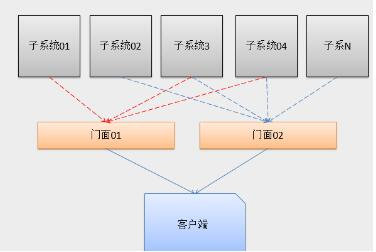# JavaScript门面模式详解

1. 门面角色

2. 子系统角色

下面我们来看一个可以提现门面模式的简单需求：主人为自己的宠物狗办理的相应的宠物领养证件

```//(定义一个静态方法来实现接口与实现类的直接检验
//静态方法不要写出Interface.prototype ,因为这是写到接口的原型链上的
//我们要把静态的函数直接写到类层次上
//定义一个接口类
var Interface=function (name,methods) {//name：接口名字
if(arguments.length<2){
}
this.name=name;
this.methods=[];//定义一个空数组装载函数名
for(var i=0;i<methods.length;i++){
if(typeof methods[i]!="string"){
}else {
this.methods.push( methods[i]);
}
}
};
Interface.ensureImplement=function (object) {
if(arguments.length<2){
throw new Error("参数必须不少于2个")
return false;
}
for(var i=1;i<arguments.length;i++){
var inter=arguments[i];
//如果是接口就必须是Interface类型
if(inter.constructor!=Interface){
throw new Error("如果是接口类的话，就必须是Interface类型");
}
//判断接口中的方法是否全部实现
//遍历函数集合
for(var j=0;j<inter.methods.length;j++){
var method=inter.methods[j];//接口中所有函数

//object[method]传入的函数
//最终是判断传入的函数是否与接口中所用函数匹配
if(!object[method]||typeof object[method]!="function" ){
//实现类中必须有方法名字与接口中所用方法名相同
throw new Error("实现类中没有完全实现接口中的所有方法")
}
}
}
}

```

（1）主人类（Person类）

```  function Person() {
this.name="测试";
this.getInfo=function () {
};
this.learn=function () {
}
this.marray=function () {
}
//验证接口
Interface.ensureImplement(this,PersonDao);//验证该类是否全部实现接口中的方法
}
```

（2）宠物狗（Dog类）

```var DogDao=new Interface("DogDao",["getInfo","call","run"]);

var Dog=function () {
this.name="gg";
this.getInfo=function () {
return "狗狗的名字"+this.name;
};
this.call=function () { };
this.run=function () {};
Interface.ensureImplement(this,DogDao);//验证接口
}

```

（3）现在可以主人可以给自己的宠物狗办理宠物领养证件了   -----客户端代码

第一种方法：不用门面的方式客户端的代码如下

```function action(person,dog) {
var r="GG"+new Date().getDay()+Math.floor(Math.random()*11);
var str="办证成功：编号"+r
+"<br/>主人信息"+person.getInfo()
+"<br>狗狗的信息："+dog.getInfo();
return str;
}document.write(action(new Person(),new Dog()));
```

#1：门面中进行如下的处理

``` function facade(person,dog) {
var r="GG"+new Date().getDay()+Math.floor(Math.random()*11);
var str="办证成功：编号"+r
+"<br/>主人信息"+person.getInfo()
+"<br>狗狗的信息："+dog.getInfo();
this.action=function () {//相当于实例的方法
return str;
};
}
```

#2：客户端负责使用的代码为

```function action2(person,dog) {